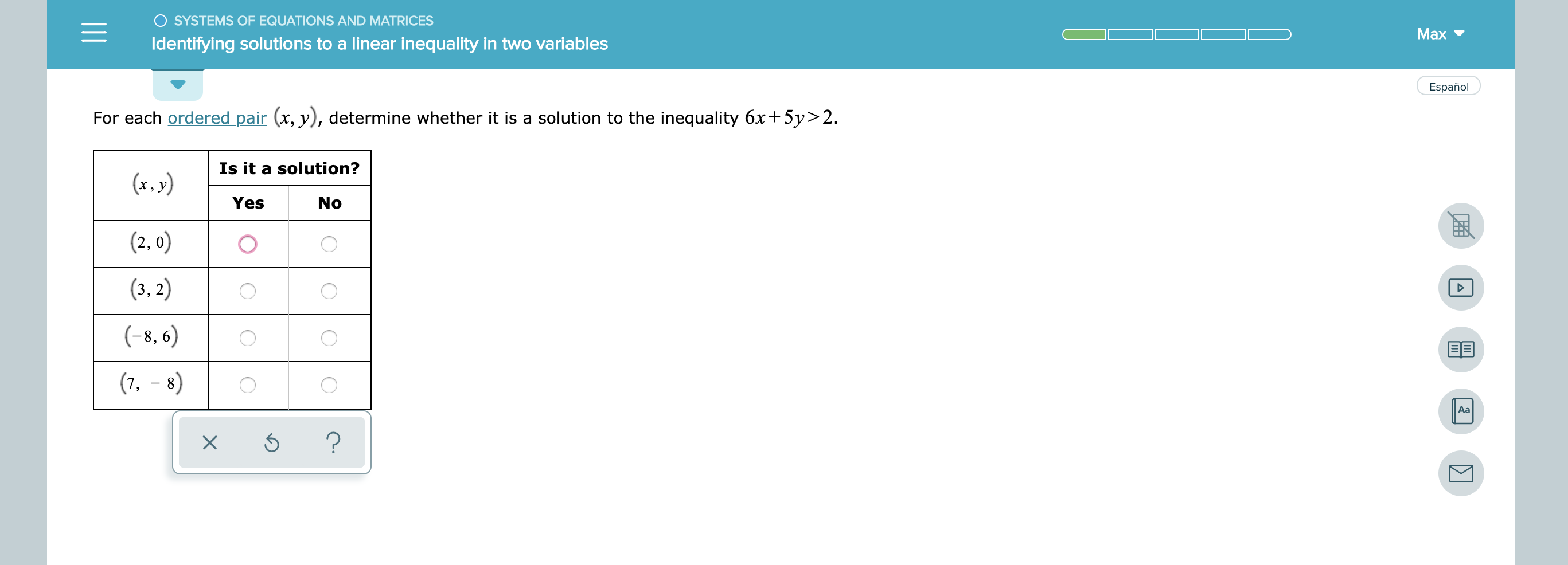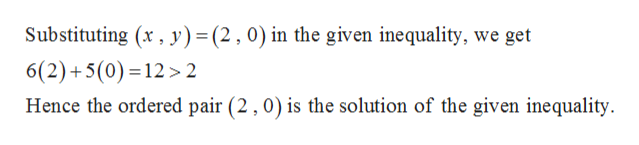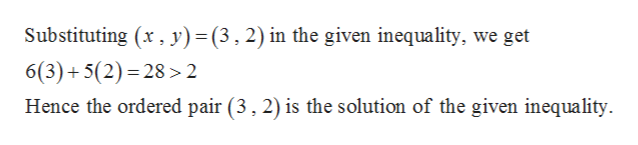# O SYSTEMS OF EQUATIONS AND MATRICESMаxIdentifying solutions to a linear inequality in two variablesEspañolFor each ordered pair (x, y), determine whether it is a solution to the inequality 6x5y> 2.Is it a solution?(x.y)YesNo(2, 0)(3, 2)(-8, 6)(7, 8)Aa?X

Question
1 views

see attachmenthelp_outlineImage TranscriptioncloseO SYSTEMS OF EQUATIONS AND MATRICES Mаx Identifying solutions to a linear inequality in two variables Español For each ordered pair (x, y), determine whether it is a solution to the inequality 6x5y> 2. Is it a solution? (x.y) Yes No (2, 0) (3, 2) (-8, 6) (7, 8) Aa ? X fullscreen
check_circle

Step 1

Given inequality is,

Step 2help_outlineImage TranscriptioncloseSubstituting (x, y) = (2,0) in the given inequality, we get 6(2)+5(0) 12> 2 Hence the ordered pair (2,0) is the solution of the given inequality fullscreen
Step 3help_outlineImage TranscriptioncloseSubstituting (x, y)= (3, 2) in the given inequality, we get 6(3)+5(2) 28>2 Hence the ordered pair (3, 2) is the solution of the given inequality fullscreen

### Want to see the full answer?

See Solution

#### Want to see this answer and more?

Solutions are written by subject experts who are available 24/7. Questions are typically answered within 1 hour.*

See Solution
*Response times may vary by subject and question.
Tagged in

### Other